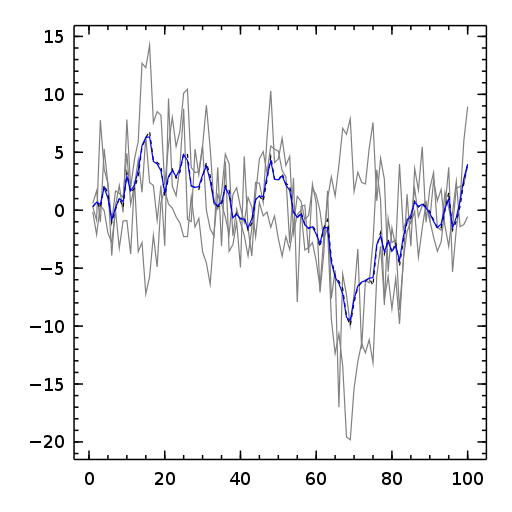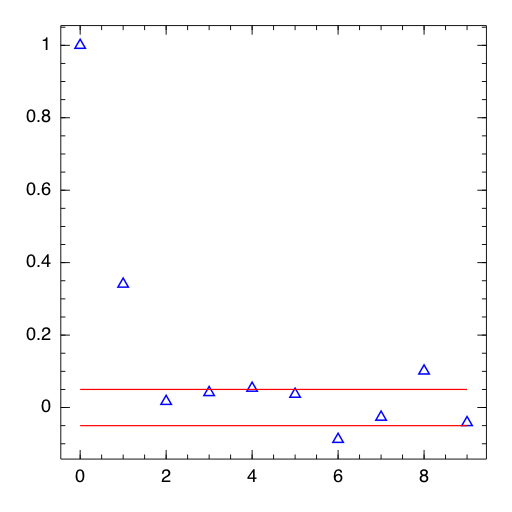40

17

24

14

# TimeModels.jl

## A Julia package for modeling time series.## GARCH model

Generalized Autoregressive Conditional Heteroskedastic (GARCH) models for Julia.

## What is implemented

• garchFit - estimates parameters of univariate normal GARCH process.
• predict - make prediction using fitted object returned by garchFit
• garchPkgTest - runs package test (compares model parameters with those obtained using R fGarch)
• Jarque-Bera residuals test
• Error analysis

Analysis of model residuals - currently only Jarque-Bera Test implemented.

## What is not ready yet

• More complex GARCH models
• Comprehensive set of residuals tests
• n-step forecasts

## Usage

### garchFit

estimates parameters of univariate normal GARCH process.

#### arguments:

data - data vector

#### returns:

Structure containing details of the GARCH fit with the following fields:

• data - orginal data
• params - vector of model parameters (omega,alpha,beta)
• llh - likelihood
• status - status of the solver
• converged - boolean convergence status, true if constraints are satisfied
• sigma - conditional volatility
• hessian - Hessian matrix
• secoef - standard errors
• tval - t-statistics

### predict

make volatility prediction

#### arguments:

fit - fitted object returned by garchFit

## Example

``````using GARCH
using Quandl
quotes = quandl("YAHOO/INDEX_GSPC")
ret = diff(log(quotes["Close"]))
ret = ret - mean(ret)
garchFit(convert(Vector,ret[end-199:end]))
``````

## References

• T. Bollerslev (1986): Generalized Autoregressive Conditional Heteroscedasticity. Journal of Econometrics 31, 307–327.
• R. F. Engle (1982): Autoregressive Conditional Heteroscedasticity with Estimates of the Variance of United Kingdom Inflation. Econometrica 50, 987–1008.

03/07/2013

21 days ago

64 commits